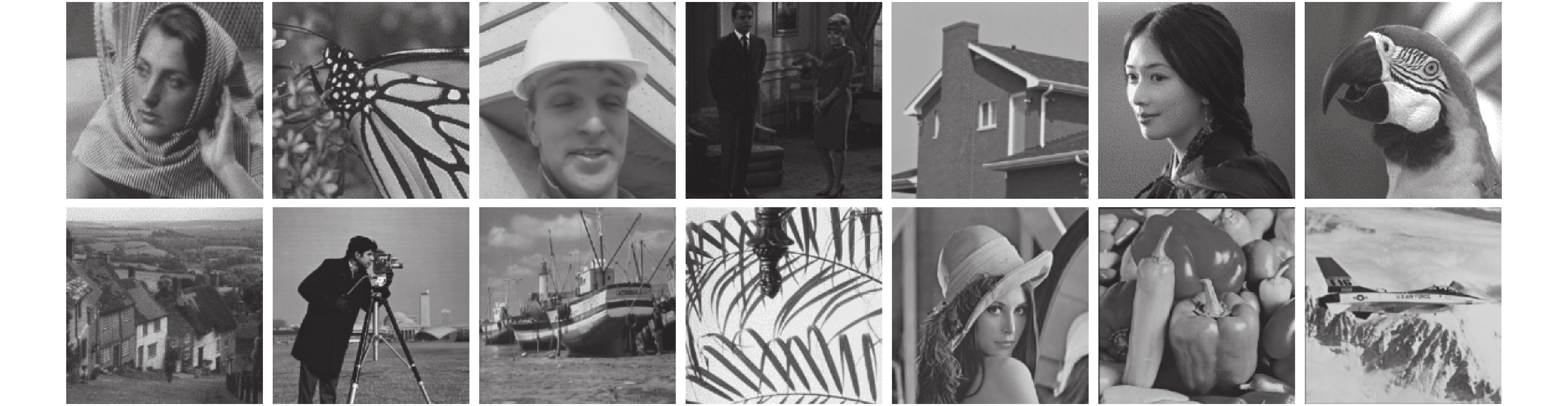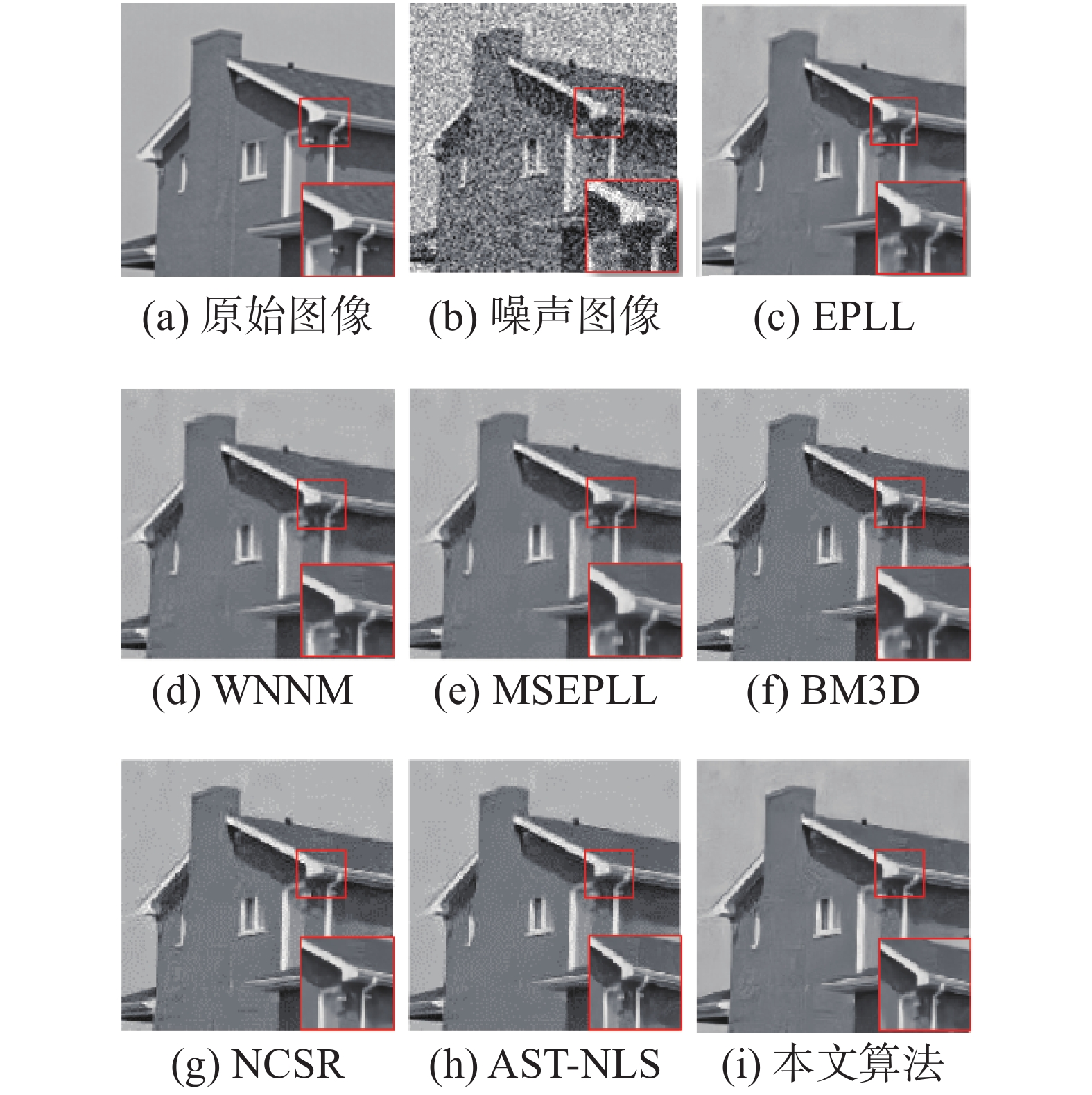﻿ 基于非凸加权<i>L<sub>p</sub></i>范数稀疏误差约束的图像去噪算法
«上一篇文章快速检索 高级检索

 智能系统学报2019, Vol. 14Issue (3): 500-507  DOI: 10.11992/tis.2018040570

### 引用本文XU Jiucheng, WANG Nan, WANG Yuyao, et al. Non-convex weighted-Lp-norm sparse-error constraint for image denoising [J]. CAAI Transactions on Intelligent Systems, 2019, 14(3): 500-507. DOI: 10.11992/tis.201804057.### 文章历史

1. 河南师范大学 计算机与信息工程学院，河南 新乡 453007;
2. 河南省高校计算智能与数据挖掘工程技术研究中心，河南 新乡 453007

Non-convex weighted-Lp-norm sparse-error constraint for image denoising
XU Jiucheng 1,2, WANG Nan 1,2, WANG Yuyao 1,2, XU Zhanwei 1,2
1. College of Computer and Information Engineering, He’nan Normal University, Xinxiang 453007, China;
2. Engineering Technology Research Center for Computing Intelligence and Data Mining in Colleges and University of He’nan Province, Xinxiang 453007, China
Abstract: Due to noise during image denoising, it is difficult to learn accurate prior knowledge. Therefore, obtaining a desirable sparse coefficient proves to be difficult. To solve this problem, this paper proposes an image denoising method based on the non-convex weighted-lp-norm sparse-error constraint. This algorithm decomposes the coefficient-solving process into two sub-problems. First, the algorithm solves the sparse coefficient in the lp norm by the generalized soft threshold value algorithm and then uses the surrogate algorithm to solve the sparse coefficient in the sparse-error constraint. Finally, the algorithm obtains a robust sparse coefficient according to its average value. The experimental results show that the proposed algorithm features a high peak signal-to-noise ratio and high efficiency in terms of the running time. Simultaneously, a desirable visual perception is obtained.
Key words: image denoising    sparse representation    sparse coefficient    prior knowledge    l1 norm    non-convex weighted lp norm    sparse error constraint    peak signal-to-noise ratio

1 基本概念

 ${N_x} = \arg \mathop {\min }\limits_{{N_i}} \sum\limits_{i = 1}^n {(\frac{1}{2}\parallel {X_i} - {D_i}{N_i}\parallel _{\rm{F}}^2} + \lambda \parallel {N_i}{\parallel _{1}})$ (1)

 ${M_y} = \arg \mathop {\min }\limits_{{M_i}} \sum\limits_{i = 1}^n {(\frac{1}{2}\parallel {Y_i} - {{\hat X}_i}\parallel _{\rm{F}}^2} + \lambda \parallel {M_i}{\parallel _{{\rm{ }}1}})$ (2)

1.1 非凸加权lp范数最小化

 ${{{M}}_y} = \arg \mathop {\min }\limits_{{{{M}}_i}} \sum\limits_{i = 1}^n {(\frac{1}{2}\parallel {Y_i} - {{\hat X}_i}\parallel _{\rm{F}}^2} + \parallel {{{W}}_i} \cdot {{{M}}_i}{\parallel _p})$

1.2 稀疏误差

 $R = M - N$

 ${M_y} = \arg \mathop {\min }\limits_{{M_i}} \sum\limits_{i = 1}^n {(\frac{1}{2}\parallel {Y_i} - \hat X\parallel _{\rm{F}}^2} + \gamma \parallel {M_i} - {N_i}{\parallel _p})$ (5)

 ${M_y} = \arg \mathop {\min }\limits_{{M_i}} \sum\limits_{i = 1}^n {(\frac{1}{2}\parallel {Y_i} - \hat X\parallel _{\rm{F}}^2} + \gamma \parallel {M_i} - {N_i}{\parallel _{{\rm{ }}1}})$
2 基于非凸加权lp范数的稀疏误差约束算法

 $\begin{array}{c} {M_y} = \arg \mathop {\min }\limits_{{M_i}} \displaystyle\sum\limits_{i = 1}^n {(\frac{1}{2}\parallel {Y_i} - \hat X\parallel _F^2} + \\ \parallel {W_i} \cdot {M_i}{\parallel _p} + \gamma \parallel {M_i} - {N_i}{\parallel _{{\rm{ }}1}}) = \\ \arg \mathop {\min }\limits_{{{{u}}_i}} (\parallel {{{y}}_i} - {{{D}}_i}{{{u}}_i}\parallel _2^2 + \parallel {{{w}}_i} \cdot {{{u}}_i}{\parallel _p} + \\ \gamma \parallel {{{u}}_i} - {{{v}}_i}{\parallel _{{\rm{ }}1}}) \end{array}$ (7)

 $\begin{array}{c} {M_y} = \arg \mathop {\min }\limits_{{{{u}}_i}} \{ \parallel {{{y}}_i} - {{{D}}_i}{{{u}}_i}\parallel _2^2 + \displaystyle\frac{1}{2}(\parallel {{{w}}_i} \cdot {{{u}}_{pi}}{\parallel _p} + \\ \gamma \parallel {{{u}}_{ri}} - {{{v}}_i}{\parallel _{{\rm{ }}1}})\} \end{array}$

 ${{{u}}_{pi}} = S_p^{{\rm{GST}}}({\delta _i};{{{w}}_i})$

 ${{{u}}_{ri}} = {S_\gamma }({{D}}_i^{ - 1}{\hat {{x}}_i} - {{{v}}_i}) + {{{v}}_i}$ (10)

1) 利用公式 ${{{Y}}^{t + 1}} = {\hat {{X}}^t} + \lambda ({{Y}} - {\hat {{X}}^t})$ 对输入的噪声图像Y进行归一化；

2) 判断Y t+1YB的结构相似性与Y tYB的结构相似性的差值是否小于阈值τ

3) 若 ${\rm{SSIM}}({{{Y}}^{t + 1}},{{{Y}}_B}) - {\rm{SSIM}}({{{Y}}^t},{{{Y}}_B}) < \tau$ ，在图像Y t+1中进行相似块选取；否则，在图像YB中进行相似块选取；

4) 通过kNN方法对每一个图像块yiYBi进行筛选，构建相似组 ${{{Y}}_i}^{t + 1}$ ${{{Y}}_{Bi}}^{t + 1}$

5) 对相似组Yi进行PCA操作，构建字典 ${{{D}}_i}^{t + 1}$

6) 由YBi=DiNiYi=Diδi分别求解第t+1次稀疏系数 ${{{N}}_i}^{t + 1}$ ${{{\delta}} _i}^{t + 1}$

7) 根据 ${{{w}}_i} = c \times 2\sqrt 2 {{{\sigma}} ^2}/{{{\sigma}} _i}$ 求解第t+1次权重 ${W_i}^{t + 1}$

8) 利用广义软阈值算法求解lp范数中的稀疏系数upi

9) 利用代理算法来求解误差约束中的稀疏系数uri

10) 通过求解upiuri的均值来计算第t+1次稀疏系数 ${{{M}}_i}^{t + 1}$

11) 根据 ${{{X}}_i}^{t + 1} = {{{D}}_i}^{t + 1}{{{M}}_i}^{t + 1}$ 求得估计图像块 ${{{X}}_i}^{t + 1}$

12) 根据加权平均合并图像块 ${{{X}}_i}^{t + 1}$ 形成去噪图像 ${\hat {{X}}^{t + 1}}$

13) 结束。

3 实验分析Download: 图 1 去噪实验的所有测试图像 Fig. 1 All test images for the denoising experiments表 1 不同去噪算法在标准差σ=20下的PSNR结果 Tab.1 Denoising PSNR results of different denoising algorithms under standard deviations σ=20表 2 不同去噪算法在标准差σ=30下的PSNR结果 Tab.2 The PSNR results of different denoising algorithms under standard deviations σ=30表 3 不同去噪算法在标准差σ=40下的PSNR结果 Tab.3 Denoising PSNR results of different denoising algorithms under standard deviations σ=40表 4 不同去噪算法在标准差σ=50下的PSNR结果 Tab.4 Denoising PSNR results of different denoising algorithms under standard deviations σ=50表 5 相关算法在14个测试图像下的平均运行时间 Tab.5 Average run time with related methods for the 14 test imagesDownload: 图 2 House测试图像的去噪结果 Fig. 2 Denoising results of House test images
4 结束语

  CHAMBOLLE A. An algorithm for total variation minimization and applications[J]. Journal of mathematical imaging and vision, 2004, 20: 89-97. DOI:10.1023/B:JMIV.0000011321.19549.88 (0)  邓承志. 图像稀疏表示理论及其应用研究[D]. 武汉: 华中科技大学, 2008. DENG Chengzhi. Research on image sparse representation theory and its applications[D]. Wuhan: Huazhong University of Science and Technology, 2008. (0)  练秋生, 张伟. 基于图像块分类稀疏表示的超分辨率重构算法[J]. 电子学报, 2012, 40(5): 920-925. LIAN Qiusheng, ZHANG Wei. Image super-resolution algorithms based on sparse representation of classified image patches[J]. Acta electronica sinica, 2012, 40(5): 920-925. (0)  DAI Tao, XU Zhiya, LIANG Haoyi, et al. A generic denoising framework via guided principal component analysis[J]. Journal of visual communication and image representation, 2017, 48: 340-352. DOI:10.1016/j.jvcir.2017.05.009 (0)  郝红侠, 刘芳, 焦李成, 等. 采用结构自适应窗的非局部均值图像去噪算法[J]. 西安交通大学学报, 2013, 47(12): 71-76. HAO Hongxia, LIU Fang, JIAO Licheng, et al. A non-local means algorithm for image denoising using structure adaptive window[J]. Journal of Xi'an jiaotong university, 2013, 47(12): 71-76. (0)  ZUO Chenglin, JOVANOV L, GOOSSENS B, et al. Image denoising using quadtree-based nonlocal means with locally adaptive principal component analysis[J]. IEEE signal processing letters, 2016, 23(4): 434-438. DOI:10.1109/LSP.2016.2530406 (0)  ZHANG Kai, ZUO Wangmeng, CHEN Yunjin, et al. Beyond a gaussian denoiser: residual learning of deep cnn for image denoising[J]. IEEE transactions on image processing, 2017, 26(7): 3142-3155. DOI:10.1109/TIP.2017.2662206 (0)  陈轶鸣, 夏景明, 陈轶才, 等. 结合稀疏表示与神经网络的医学图像融合[J]. 河南科技大学学报(自然科学版), 2018, 39(2): 40-47. CHEN Yiming, XIA Jingming, CHEN Yicai, et al. Medical image fusion combining sparse representation and neural network[J]. Journal of Henan University of Science and Technology (natural science), 2018, 39(2): 40-47. (0)  解凯, 张芬. 基于过完备表示的图像去噪算法[J]. 电子学报, 2013, 41(10): 1911-1916. XIE Kai, ZHANG Fen. Overcomplete representation base image denoising algorithm[J]. Acta electronica sinica, 2013, 41(10): 1911-1916. DOI:10.3969/j.issn.0372-2112.2013.10.006 (0)  BUDIANTO, LUN D P. Robust fringe projection profilometry via sparse representation[J]. IEEE transactions on image processing, 2016, 25(4): 1726-1739. DOI:10.1109/TIP.2016.2530313 (0)  ZHANG Jian, ZHAO Debin, GAO Wen. Group-based sparse representation for image restoration[J]. IEEE transactions on image processing, 2014, 23(8): 3336-3351. DOI:10.1109/TIP.2014.2323127 (0)  占美全, 邓志良. 基于L1范数的总变分正则化超分辨率图像重建 [J]. 科学技术与工程, 2010, 10(28): 6903-6906. ZHAN Meiquan, DENG Zhiliang. L1 norm of total variation regularization based super resolution reconstruction for images [J]. Science technology and engineering, 2010, 10(28): 6903-6906. DOI:10.3969/j.issn.1671-1815.2010.28.011 (0)  杨平先, 陈明举. 一种基于L1范数的非局部变分图像复原模型 [J]. 液晶与显示, 2017, 32(8): 635-641. YANG Pingxian, CHEN Mingju. A nonlocal total variation based on L1 norm for image recovery [J]. Chinese journal of liquid crystals and displays, 2017, 32(8): 635-641. (0)  张艳艳, 陈苏婷, 葛俊祥, 等. 自适应非凸稀疏正则化下自适应光学系统加性噪声的去除[J]. 物理学报, 2017, 66(12): 368-375. ZHANG Yanyan, CHEN Suting, GE Junxiang, et al. Removal of additive noise in adaptive optics system based on adaptive nonconvex sparse regularization[J]. Acta physica sinica, 2017, 66(12): 368-375. DOI:10.7498/aps.66.129501 (0)  ZHA Zhiyuan, LIU Xin, HUANG Xiaohua, et al. Analyzing the group sparsity based on the rank minimization methods[C]//Proceedings of IEEE International Conference on Multimedia and Expo. Hong Kong, China, 2017: 883–888. (0)  ZUO Wangmeng, MENG Deyu, ZHANG Lei, et al. A generalized iterated shrinkage algorithm for non-convex sparse coding[C]//Proceedings of IEEE International Conference on Computer Vision. Sydney, NSW, Australia, 2013: 217–224. (0)  ZHANG Xiaoqun, BURGER M, BRESSON X, et al. Bregmanized nonlocal regularization for deconvolution and sparse reconstruction[J]. SIAM journal on imaging sciences, 2010, 3(3): 253-276. DOI:10.1137/090746379 (0)  DONG Weisheng, ZHANG Lei, SHI Guangming, et al. Nonlocally centralized sparse representation for image restoration[J]. IEEE transactions on image processing, 2013, 22(4): 1620-1630. DOI:10.1109/TIP.2012.2235847 (0)  GU Shuhang, XIE Qi, MENG Deyu, et al. Weighted nuclear norm minimization and its applications to low level vision[J]. International journal of computer vision, 2017, 121(2): 183-208. DOI:10.1007/s11263-016-0930-5 (0)  LUO Enming, CHAN S H, NGUYEN T Q. Adaptive image denoising by mixture adaptation[J]. IEEE transactions on image processing, 2016, 25(10): 4489-4503. DOI:10.1109/TIP.2016.2590318 (0)  LIU Hangfan, XIONG Ruiqin, ZHANG Jian, et al. Image denoising via adaptive soft-thresholding based on non-local samples[C]//Proceedings of IEEE Conference on Computer Vision and Pattern Recognition. Boston, MA, USA, 2015: 484–492. (0)  PAPYAN V, ELAD M. Multi-scale patch-based image restoration[J]. IEEE transactions on image processing, 2016, 25(1): 249-261. DOI:10.1109/TIP.2015.2499698 (0)